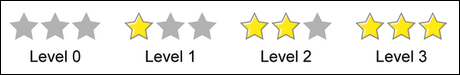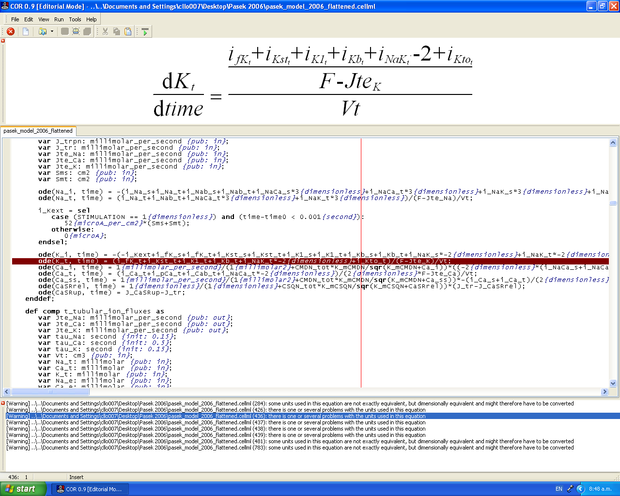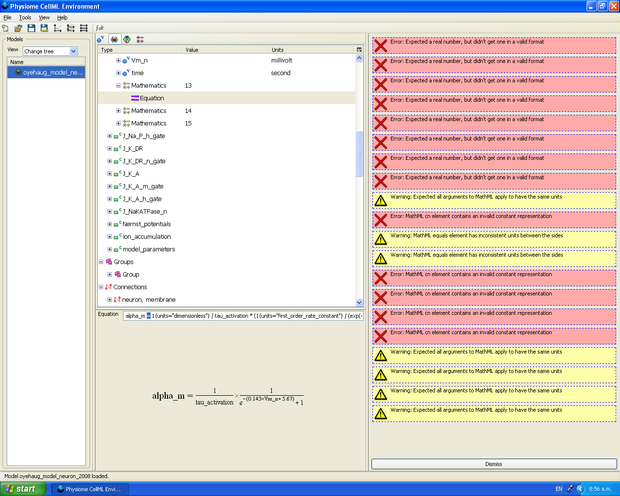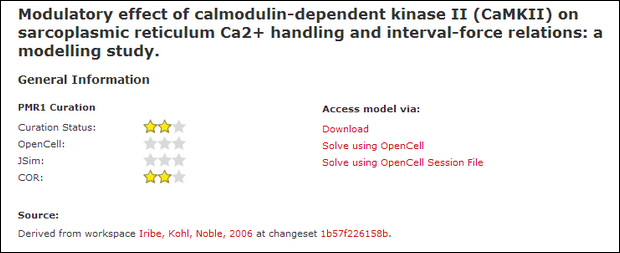# Curating models in the CellML model repository

This document is not so much a tutorial, as it is an outline of theory underlining CellML model curation and the steps we go through to fix a model. Rather than advocating these as "best practice", we regard them as a compromise, but the best current solution. Our future plans are outlined in the appropriate sections below.

## 2. CellML model curation: the theory

The basic measure of curation for a CellML model is described by the curation level of the model document. We have defined four levels of curation:

• Level 0: not curated;
• Level 1: the CellML model description is consistent with the mathematics in the original published paper;
• Level 2: the CellML model has been checked for:
• (i) typographical errors,
• (ii) consistency of units,
• (iii) completeness; such that all parameters and initial conditions have been defined,
• (iv) over-constraints; such that the model does not contain equations or initial values which are either redundant or inconsistent, and
• (v) consistency of simulation output with the published results; such that running the model in an appropriate simulation environment reproduces the results in the original paper;
• Level 3: the model has been checked to the extent that it is known to satisfy physical constraints such as conservation of mass, momentum, charge, etc. This level of curation needs to be conducted by specialised domain experts.

In the repository, a 'star' system signifies the curation status of a CellML model:However, from experience, we have found that levels 1 and 2 are often mutually exclusive. That is, in order to get the CellML model to replicate the published results (level 2) we have to adjust the published model description (level 1). Frequently, the errors introduced into the model during the publication process require us to correct minor typographical errors or unit inconsistencies, and/or contact the original model author to request missing parameter values or equations.

Further, it is our experience that the current system of counting the number of stars can lead to uncertainty as to how to interpret the curation status of a model. We suggest a more descriptive set of "curation flags" may be more useful. Such "flags" could include "runs in PCEnv", "units/dimensions consistent", "gives same results as referenced publication", etc. The exact method of implementing these flags has yet to be determined. Until then, the star system remains the compromise method of assigning a curation status to a model.

## 3. CellML model curation: the practice

Of the several hundred models in the repository, over half have been curated to some degree. Our goal is to complete the curation of all the models in the repository, ideally to the level that they replicate the results in the published paper (level 2).

The process of model curation involves the following sequence of actions:

• The CellML model is loaded into an editing and simulation environment such as Opencell, or Cellular Open Resource (COR). Any obvious typographical errors and unit inconsistencies are corrected, which is facilitated by a series of error messages and validation prompts generated by the software, and the rendering of the MathML equations in an easily readable format.• Assuming the model runs, we then compare the simulation output with the results in the published paper, which typically involves comparing the graphical results with the published figures in the original paper.
• If we cannot get the CellML model to run, or the simulation output disagrees with the published results, we then attempt to contact the original model author(s) and seek their advice and, where possible, obtain the original model code, which may be in a wide range of different programming languages.

Ultimately, we would like to encourage the scientific modeling community - including model authors, journals and publishing houses - to publish their models in CellML concurrent with the publication of the printed article. This will eliminate the need for code-to-text-to-code translations and thus avoid many of the errors which are introduced during the translation process.

## 4. CellML model simulation curation status: theory and practice

As part of the process of model curation, it is important to know what tools were used to run the model and how well the model runs in a specific simulation environment. In this case, the theory and the practice are essentially the same thing, and we carry out a series of simulation steps which then translate into a confidence level as part of a simulator's metadata for each model. The four confidence levels are defined as:

• Level 0: not curated;
• Level 1: the model loads and runs in the specified simulation environment;
• Level 2: the model produces results that are qualitatively similar to those previously published for the model;
• Level 3: the model has been quantitatively and rigorously verified as producing identical results to the original published model.

As with the general curation status of the model, again we use a star system to indicate the model simulation curation level for each of three simulation environments.Curation status indicators as they appear on the repository page for a model.